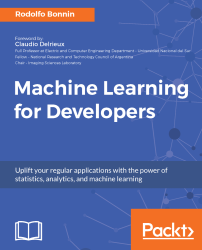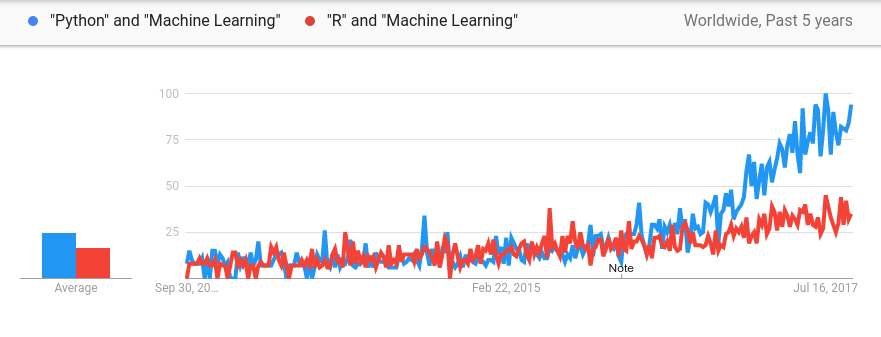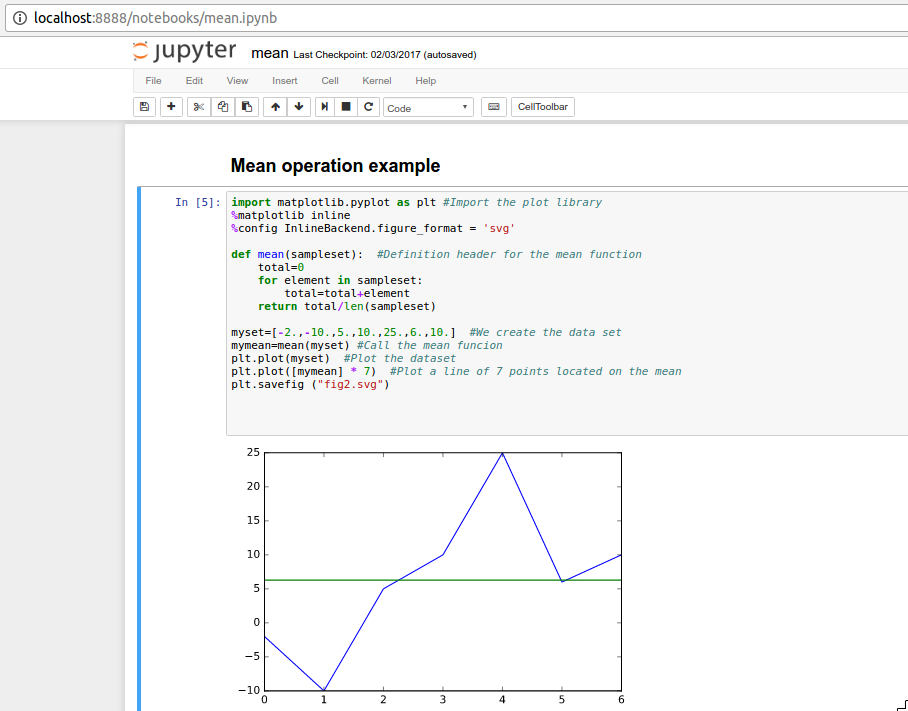•#### Machine Learning for Developers#### Overview of this book

Most of us have heard about the term Machine Learning, but surprisingly the question frequently asked by developers across the globe is, “How do I get started in Machine Learning?”. One reason could be attributed to the vastness of the subject area because people often get overwhelmed by the abstractness of ML and terms such as regression, supervised learning, probability density function, and so on. This book is a systematic guide teaching you how to implement various Machine Learning techniques and their day-to-day application and development. You will start with the very basics of data and mathematical models in easy-to-follow language that you are familiar with; you will feel at home while implementing the examples. The book will introduce you to various libraries and frameworks used in the world of Machine Learning, and then, without wasting any time, you will get to the point and implement Regression, Clustering, classification, Neural networks, and more with fun examples. As you get to grips with the techniques, you’ll learn to implement those concepts to solve real-world scenarios for ML applications such as image analysis, Natural Language processing, and anomaly detections of time series data. By the end of the book, you will have learned various ML techniques to develop more efficient and intelligent applications.
PrefaceFree Chapter
Introduction - Machine Learning and Statistical ScienceThe Learning ProcessClusteringLinear and Logistic RegressionNeural NetworksConvolutional Neural NetworksRecurrent Neural NetworksRecent Models and DevelopmentsSoftware Installation and Configuration# Tools of the trade–programming language and libraries

As this book is aimed at developers, we think that the approach of explaining the mathematical concepts using real code comes naturally.

When choosing the programming language for the code examples, the first approach was to use multiple technologies, including some cutting-edge libraries. After consulting the community, it was clear that a simple language would be preferable when explaining the concepts.

Among the options, the ideal candidate would be a language that is simple to understand, with real-world machine learning adoption, and that is also relevant.

The clearest candidate for this task was Python, which fulfils all these conditions, and especially in the last few years has become the go-to language for machine learning, both for newcomers and professional practitioners.

In the following graph, we compare the previous star in the machine learning programming language field, R, and we can clearly conclude the huge, favorable tendency towards using Python. This means that the skills you acquire in this book will be relevant now and in the foreseeable future:Interest graph for R and Python in the Machine Learning realm.

In addition to Python code, we will have the help of a number of the most well-known numerical, statistical, and graphical libraries in the Python ecosystem, namely pandas, NumPy, and matplotlib. For the deep neural network examples, we will use the Keras library, with TensorFlow as the backend.

# The Python language

Python is a general-purpose scripting language, created by the Dutch programmer Guido Van Rossum in 1989. It possesses a very simple syntax with great extensibility, thanks to its numerous extension libraries, making it a very suitable language for prototyping and general coding. Because of its native C bindings, it can also be a candidate for production deployment.

The language is actually used in a variety of areas, ranging from web development to scientific computing, in addition to its use as a general scripting tool.

# The NumPy library

If we had to choose a definitive must-use library for use in this book, and a non-trivial mathematical application written in Python, it would have to be NumPy. This library will help us implement applications using statistics and linear algebra routines with the following components:

• A versatile and performant N-dimensional array object
• Many mathematical functions that can be applied to these arrays in a seamless manner
• Linear algebra primitives
• Random number distributions and a powerful statistics package
• Compatibility with all the major machine learning packages
The NumPy library will be used extensively throughout this book, using many of its primitives to simplify the concept explanations with code.

# The matplotlib library

Data plotting is an integral part of data science and is normally the first step an analyst performs to get a sense of what's going on in the provided set of data.

For this reason, we need a very powerful library to be able to graph the input data, and also to represent the resulting output. In this book, we will use Python's matplotlib library to describe concepts and the results from our models.

# What's matplotlib?

Matplotlib is an extensively used plotting library, especially designed for 2D graphs. From this library, we will focus on using the pyplot module, which is a part of the API of matplotlib and has MATLAB-like methods, with direct NumPy support. For those of you not familiar with MATLAB, it has been the default mathematical notebook environment for the scientific and engineering fields for decades.

The method described will be used to illustrate a large proportion of the concepts involved, and in fact, the reader will be able to generate many of the examples in this book with just these two libraries, and using the provided code.

# Pandas

Pandas complements the previously mentioned libraries with a special structure, called DataFrame, and also adds many statistical and data mangling methods, such as I/O, for many different formats, such as slicing, subsetting, handling missing data, merging, and reshaping, among others.

The DataFrame object is one of the most useful features of the whole library, providing a special 2D data structure with columns that can be of different data types. Its structure is very similar to a database table, but immersed in a flexible programming runtime and ecosystem, such as SciPy. These data structures are also compatible with NumPy matrices, so we can also apply high-performance operations to the data with minimal effort.

# SciPy

SciPy is a stack of very useful scientific Python libraries, including NumPy, pandas, matplotlib, and others, but it also the core library of the ecosystem, with which we can also perform many additional fundamental mathematical operations, such as integration, optimization, interpolation, signal processing, linear algebra, statistics, and file I/O.

# Jupyter notebook

Jupyter is a clear example of a successful Python-based project, and it's also one of the most powerful devices we will employ to explore and understand data through code.

Jupyter notebooks are documents consisting of intertwined cells of code, graphics, or formatted text, resulting in a very versatile and powerful research environment. All these elements are wrapped in a convenient web interface that interacts with the IPython interactive interpreter.

Once a Jupyter notebook is loaded, the whole environment and all the variables are in memory and can be changed and redefined, allowing research and experimentation, as shown in the following screenshot:Jupyter notebook

This tool will be an important part of this book's teaching process, because most of the Python examples will be provided in this format. In the last chapter of the book, you will find the full installation instructions.

After installing, you can cd into the directory where your notebooks reside, and then call Jupyter by typing jupyter notebook